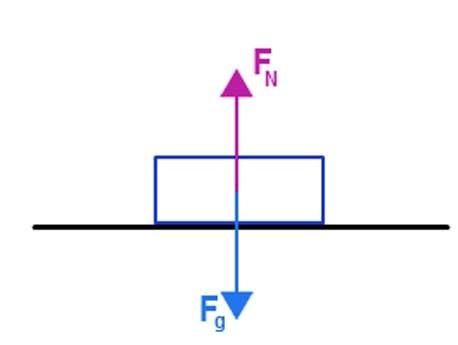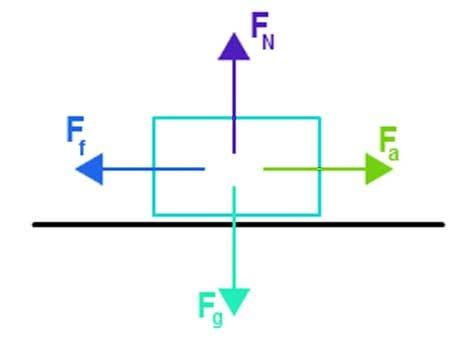# Net Force Formula

The net force is defined as is the sum of all the forces acting on an object. Net force can accelerate a mass. Some other force acts on a body either at rest or motion. The net force is a term used in a system when there is a significant number of forces.

## Formula of Net Force

If N is the number of forces acting on a body, the net force formula is given by,

FNet = F1 + F2 + F3….+ FN

Where,

F1, F2, F3…FN is the force acting on a body.

Net force when a body is at rest:When the body is at rest, the net force formula is given by,

FNet = Fa + Fg.

Where,

Fa = applied force,

Fg = gravitational force.
Net force when a body is in motion:When a force is applied to the body, not only is the applied force acting, there are many other forces like gravitational force  Fg, frictional force Ff and the normal force that balances the other force.

Therefore, the net force formula is given by,

FNet = Fa + Fg + Ff + FN.

Where,

• Fa is applied force,
• Fg is the gravitational force,
• Ff is the frictional force,
• FN is a normal force.

### Examples of Net Force

Example 1

In a tug of war, a fat man pulls with a force of 100 N on a side, and a lean man pulls with 90 N on the other side. Determine the net force.

Solution:

Given:

Force F1= 100 N

Force F2 = -90 N

The net force formula is given by

FNet = F1 + F2

FNet  = 100 – 90

FNet  = 10 N

Therefore, the net force is 10 N.

Example 2

A toy car is at rest, and a force of 70 N is applied to it. If the frictional force of 20 N, determine the net force.

Solution:

Given:

Applied force Fa = 70 N

Frictional force Ff = -20 N

The net force formula is given by

FNet = Fa + Ff

FNet = 70 – 20

FNet  = 50 N

Therefore, the net force is 50 N